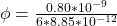## A charge of 0.80 nC is placed at the center of a cube that measures 4.0 m along each edge. What is the electric flux through one face of the

Question

A charge of 0.80 nC is placed at the center of a cube that measures 4.0 m along each edge. What is the electric flux through one face of the cube

in progress 0
5 months 2021-08-14T05:28:26+00:00 1 Answers 40 views 0

## Answers ( )

The magnetic  flux is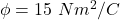Explanation:

From the question we are told that

The value of the charge is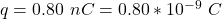The length of each side of  the cube is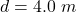Generally the magnetic flux through a closed surface is  mathematically represented as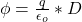Where  D is the area enclosing the charge

Now  a  cube is made up of six faces but in this question we are considering only one face which is mathematically represented as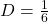So  the electric flux through one face of the cube  is  mathematically represented as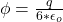where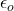is the permitivity of free space with value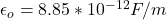substituting value